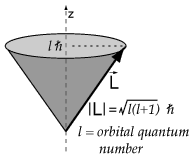# Question 522dd

Feb 17, 2018

$1.310976 \times {10}^{-} 23 \text{J/T}$

#### Explanation:

The magnetic orbital moment is given by

mu_"orb" = -g_"L"(e/(2m_e)"L")#

where $\text{L}$ is orbital angular momentum

$| \text{L} | = \sqrt{l \left(l + 1\right)} \frac{h}{2 \pi}$${g}_{L}$ is the electron orbital g- factor which is equal to 1

$l$ for a the ground state orbital or 1s orbital is 0

so the magnetic orbital moment is also 0$l$ for the 4p orbital is 1

${\mu}_{\text{orb" = -g_"L}} \left(\frac{e}{2 {m}_{e}} \sqrt{l \left(l + 1\right)} \frac{h}{2 \pi}\right)$

${\mu}_{\text{orb" = -g_"L}} \left(\frac{e}{2 {m}_{e}} \sqrt{1 \left(1 + 1\right)} \frac{h}{2 \pi}\right)$

A unit of magnetic moment called the "Bohr magneton" is introduced here.

${\mu}_{\text{B" = (ebarh)/(2m_e) ~~ 9.27xx10^24"J/T}}$

$\overline{h} = \frac{h}{2 \pi}$

$\frac{e}{2 {m}_{e}} = {\mu}_{\text{B}} / \overline{h}$

${\mu}_{\text{orb" = -g_"L}} \left(\frac{e}{2 {m}_{e}} \sqrt{1 \left(1 + 1\right)} \frac{h}{2 \pi}\right)$

$= - {\mu}_{\text{B}} \sqrt{2}$

$- 9.27 \times {10}^{-} 24 \text{J/T} \times \sqrt{2}$

$\approx - 9.27 \times {10}^{-} 24 \text{J/T} \times 1.41421356237$

$- 1.310976 \times {10}^{-} 23 \text{J/T}$

So the difference between them is
$1.310976 \times {10}^{-} 23 \text{J/T}$